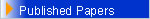TeachingI. Undergraduate Program Theory of Probability and Statistics. Random experiment and Sample space. Classical and conditional probability. Bayes' formulas. Random variable. Stochastic function and Probability distributions. Normal distribution. Mathematics III. Limits, derivatives, simple integrals and applications. Partial differentiation. Extrema subject to constraints. Method of Lagrange Multipliers. Number Theory. Numbers and Divisibility. Diophantine equations. Congruences. Mathematical computation of the Orthodox Easter by Gauss. Mathematical Programming. Gauss-Jordan elimination method. Geometric Method. Simplex Method. Duality principle and dual problem by John von Neumann. Applications Mathematical Topics. Continued fractions. Fibonacci numbers and recursive sequences. Lucas numbers. Golden-section number. Applications. Center of gravity and centroid. Fractions,equations and analysis in Music. II. Graduate Program Analysis. Multiple integration. Functional equations and inequalities, Ulam stability problem. Combinatorial Analysis and Computational Mathematics. Combinations and Permutations.Applications. Differential equations. Mixed type equations and Tricomi boundary value problem. Tutorial Analysis , Classical inequalities. Landau differential inequalities and Heisenberg integral inequalities. History of Analysis, Famous Problems and Theorems with their History.